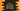# How to use std::move in C++ STL explanation with example## std::move in C++ STL example:

std::move is defined in algorithm header. It moves the elements in a range into a different iterator.

In this post, we will learn how to use std::move with example in C++.

### Definition:

std::move is defined as below:

``OutputIterator move (InputIterator first, InputIterator last, OutputIterator result)``

Here,

• first and second are the initial and final positions of the sequence. It includes all the elements including the element pointed by first, but not the element pointed by last.
• result is the output iterator to the initial position in the result sequence.

### Return value:

It returns an iterator to the end of the destination range where elements are moved.

### Example :

Let’s take a look at the below example:

``````#include <iostream>
#include <algorithm>
#include <vector>

using namespace std;

int main()
{
int inputArr[] = {1, 2, 3, 4, 5, 6, 7, 8, 9, 10};
vector<int> output(5);

copy(inputArr + 3, inputArr + 8, output.begin());

for (int i : output)
cout << i << endl;
}``````

It will print the below output:

``````4
5
6
7
8``````

It moves all items from inputArr to inputArr to the vector output.